Type
Quiz
Book Title
Fundamentals of Corporate Finance Standard Edition 9th Edition
ISBN 13
978-0073382395

### 978-0073382395 Chapter 16 Questions and Problems 1-10

April 3, 2019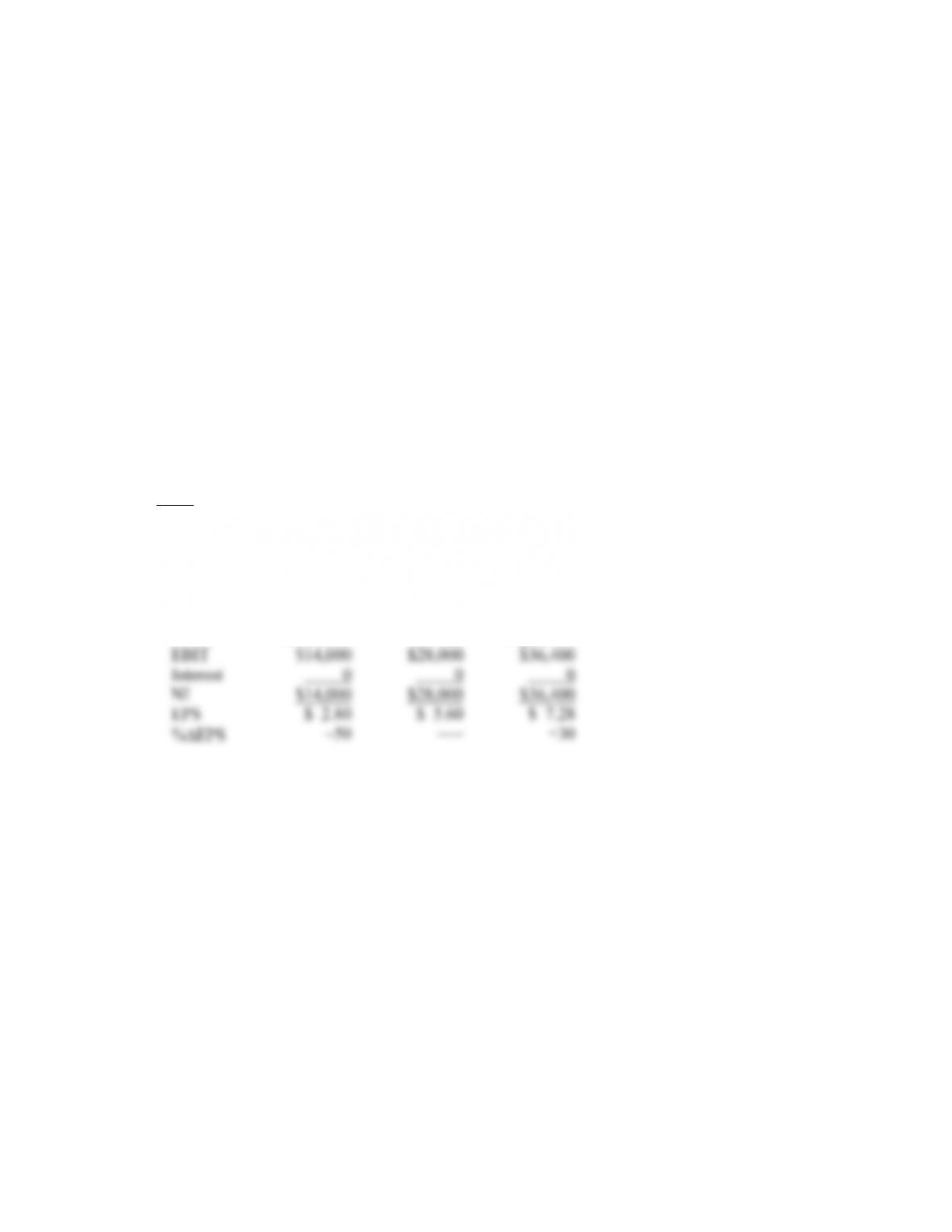CHAPTER 16 B-277
9. One side is that Continental was going to go bankrupt because its costs made it uncompetitive. The
bankruptcy filing enabled Continental to restructure and keep flying. The other side is that Continental
abused the bankruptcy code. Rather than renegotiate labor agreements, Continental simply abrogated
them to the detriment of its employees. In this, and the last several, questions, an important thing to
keep in mind is that the bankruptcy code is a creation of law, not economics. A strong argument can
always be made that making the best use of the bankruptcy code is no different from, for example,
minimizing taxes by making best use of the tax code. Indeed, a strong case can be made that it is the
financial manager’s duty to do so. As the case of Continental illustrates, the code can be changed if
socially undesirable outcomes are a problem.
10. The basic goal is to minimize the value of non-marketed claims.
Solutions to Questions and Problems
NOTE: All end of chapter problems were solved using a spreadsheet. Many problems require multiple steps.
Due to space and readability constraints, when these intermediate steps are included in this solutions
manual, rounding may appear to have occurred. However, the final answer for each problem is found
without rounding during any step in the problem.
Basic
1. a. A table outlining the income statement for the three possible states of the economy is shown
below. The EPS is the net income divided by the 5,000 shares outstanding. The last row shows the
percentage change in EPS the company will experience in a recession or an expansion economy.
Recession Normal Expansion
b. If the company undergoes the proposed recapitalization, it will repurchase:
Share price = Equity / Shares outstanding
Share price = \$250,000/5,000
Share price = \$50
Shares repurchased = Debt issued / Share price
Shares repurchased =\$90,000/\$50
Shares repurchased = 1,800B-278 SOLUTIONS
2. a. A table outlining the income statement with taxes for the three possible states of the economy is
shown below. The share price is still \$50, and there are still 5,000 shares outstanding. The last row
shows the percentage change in EPS the company will experience in a recession or an expansion
economy.
b. A table outlining the income statement with taxes for the three possible states of the economy and
assuming the company undertakes the proposed capitalization is shown below. The interest
payment and shares repurchased are the same as in part b of Problem 1.
Recession Normal Expansion
EBIT \$14,000 \$28,000 \$36,400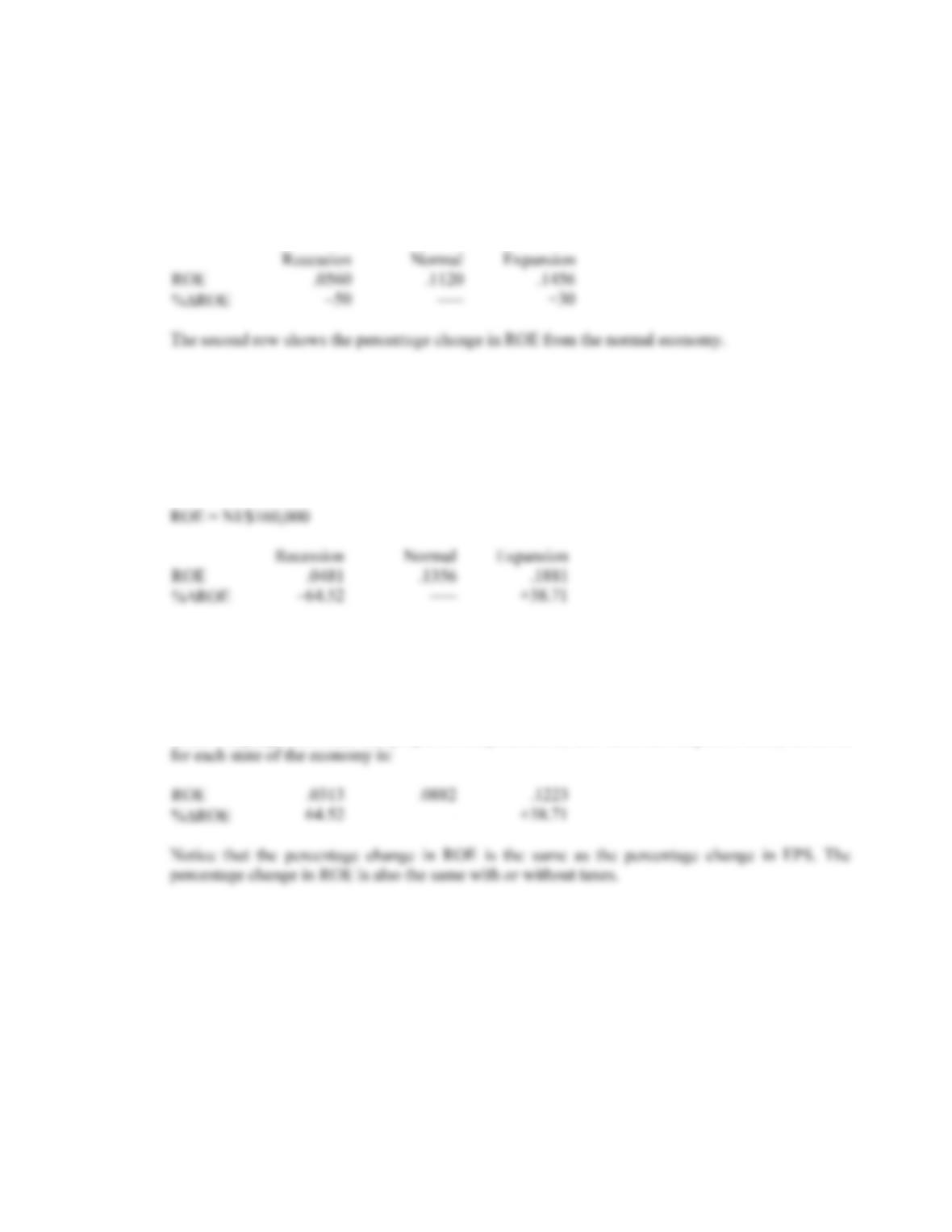CHAPTER 16 B-279
3. a. Since the company has a market-to-book ratio of 1.0, the total equity of the firm is equal to the
market value of equity. Using the equation for ROE:
ROE = NI/\$250,000
The ROE for each state of the economy under the current capital structure and no taxes is:
b. If the company undertakes the proposed recapitalization, the new equity value will be:
Equity = \$250,000 – 90,000
Equity = \$160,000
So, the ROE for each state of the economy is:
c. If there are corporate taxes and the company maintains its current capital structure, the ROE is:
ROE .0364 .0728 .0946
%ROE –50 ––– +30
If the company undertakes the proposed recapitalization, and there are corporate taxes, the ROE
4. a. Under Plan I, the unlevered company, net income is the same as EBIT with no corporate tax. The
EPS under this capitalization will be:
EPS = \$350,000/160,000 shares
EPS = \$2.19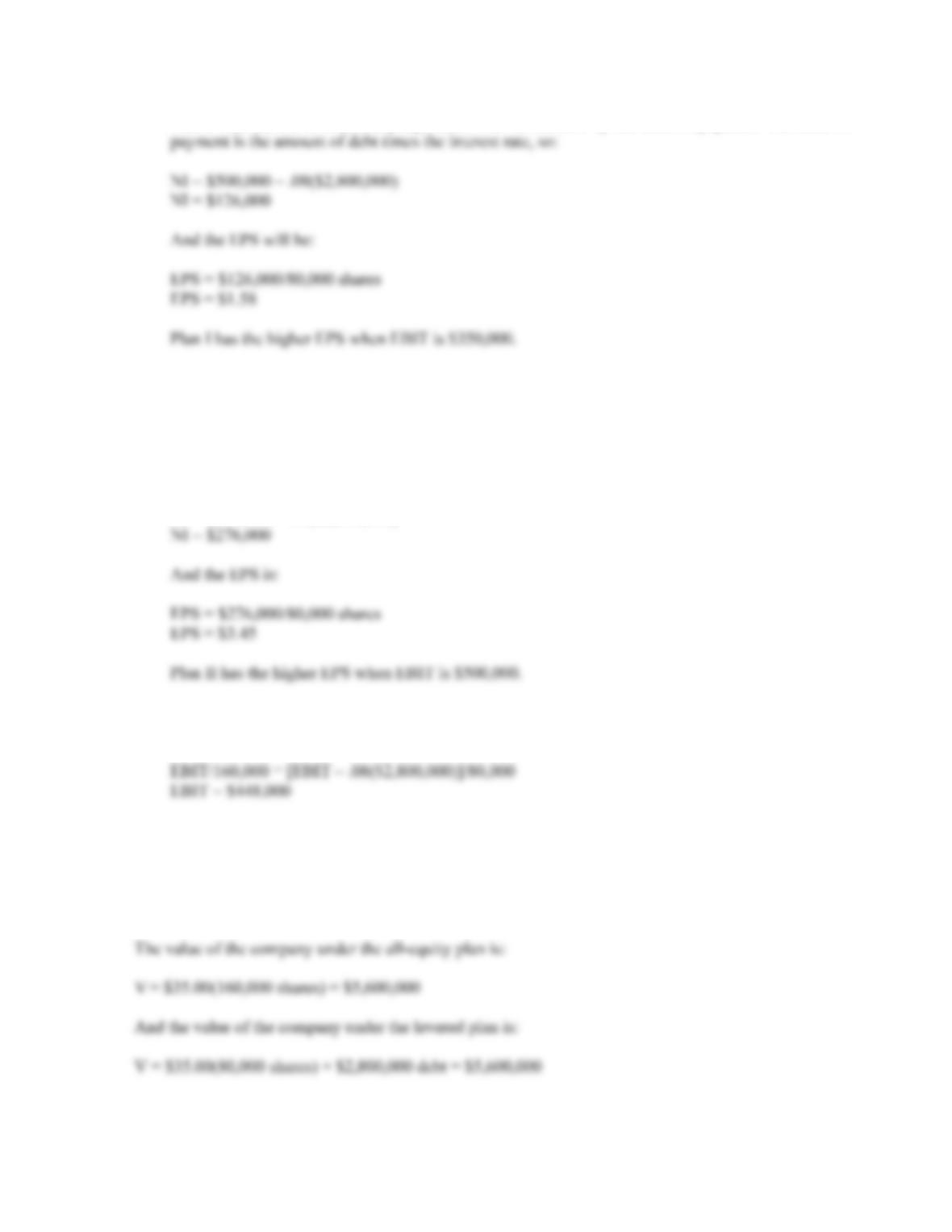B-280 SOLUTIONS
Under Plan II, the levered company, EBIT will be reduced by the interest payment. The interest
b. Under Plan I, the net income is \$500,000 and the EPS is:
EPS = \$500,000/160,000 shares
EPS = \$3.13
Under Plan II, the net income is:
NI = \$500,000 – .08(\$2,800,000)
c. To find the breakeven EBIT for two different capital structures, we simply set the equations for
EPS equal to each other and solve for EBIT. The breakeven EBIT is:
5. We can find the price per share by dividing the amount of debt used to repurchase shares by the number
of shares repurchased. Doing so, we find the share price is:
Share price = \$2,800,000/(160,000 – 80,000)
Share price = \$35.00 per share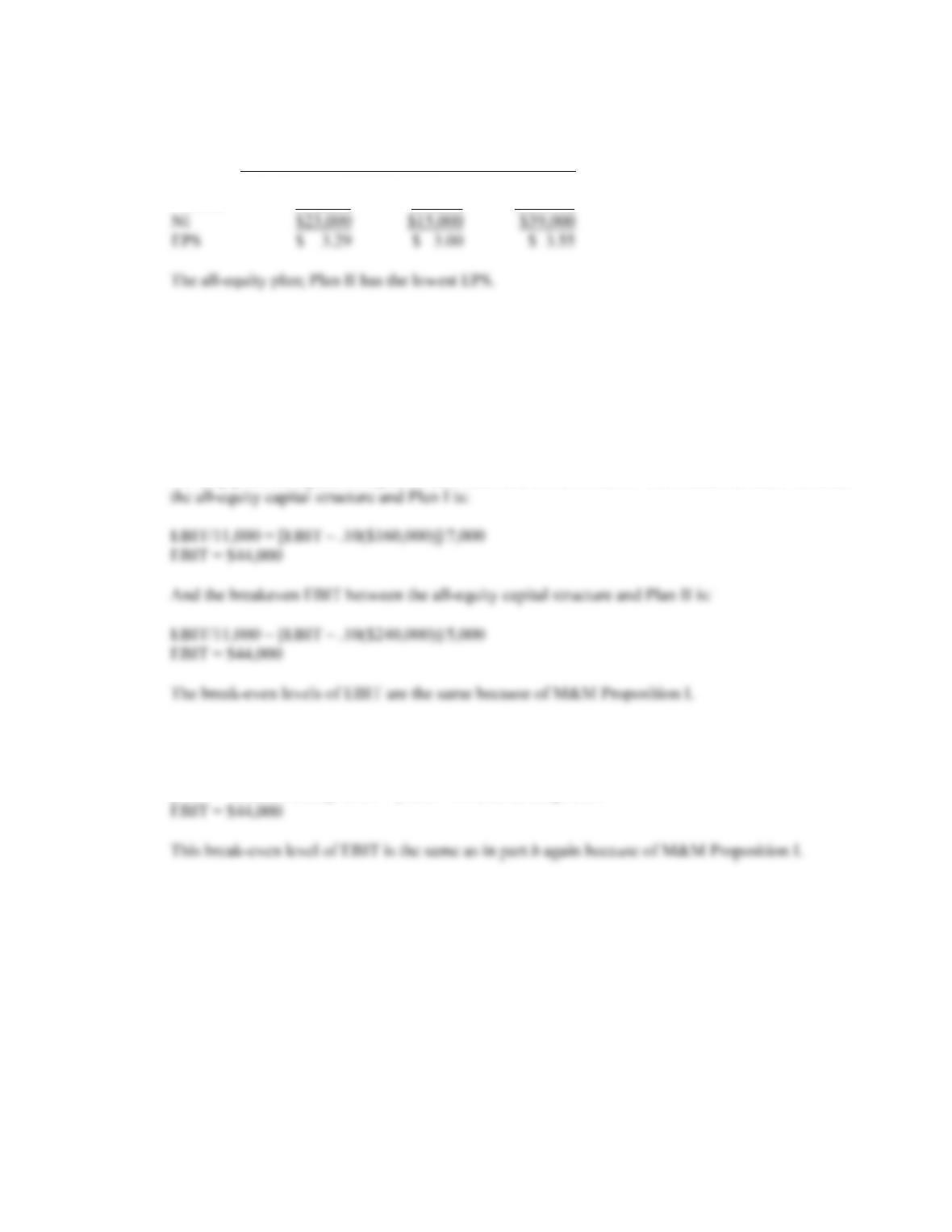CHAPTER 16 B-281
6. a. The income statement for each capitalization plan is:
I
I
I
All-equity
EBIT \$39,000 \$39,000 \$39,000
Interest 16,000 24,000 0
b. The breakeven level of EBIT occurs when the capitalization plans result in the same EPS. The EPS
is calculated as:
EPS = (EBIT – RDD)/Shares outstanding
This equation calculates the interest payment (RDD) and subtracts it from the EBIT, which results
in the net income. Dividing by the shares outstanding gives us the EPS. For the all-equity capital
structure, the interest term is zero. To find the breakeven EBIT for two different capital structures,
we simply set the equations equal to each other and solve for EBIT. The breakeven EBIT between
c. Setting the equations for EPS from Plan I and Plan II equal to each other and solving for EBIT, we
get:
[EBIT – .10(\$160,000)]/7,000 = [EBIT – .10(\$240,000)]/5,000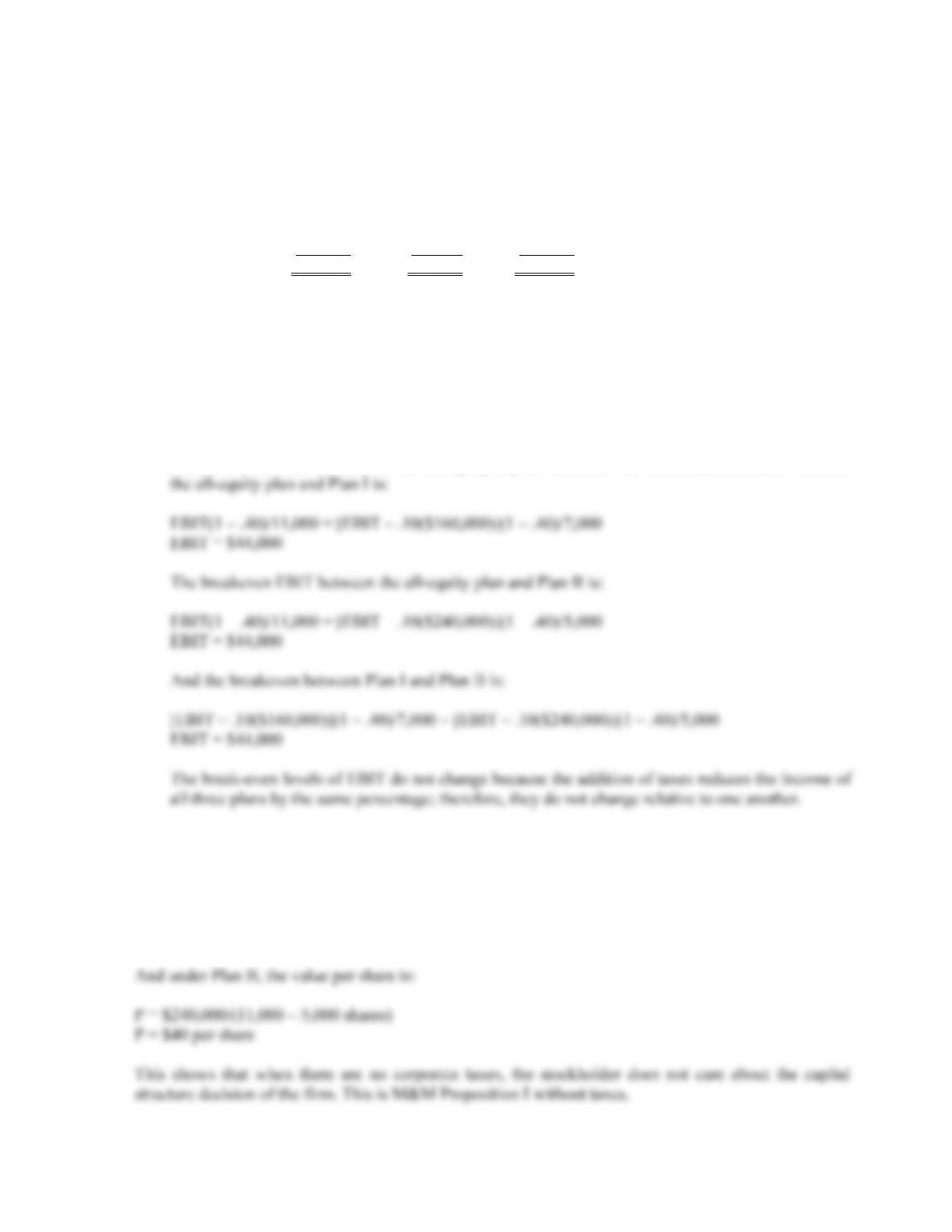B-282 SOLUTIONS
d. The income statement for each capitalization plan with corporate income taxes is:
I
I
I
All-equity
EBIT \$39,000 \$39,000 \$39,000
Interest 16,000 24,000 0
Taxes 9,200 6,000 15,600
NI \$ 13,800 \$ 9,000 \$ 23,400
EPS \$ 1.97 \$ 1.80 \$ 2.13
The all-equity plan still has the highest EPS; Plan II still has the lowest EPS.
We can calculate the EPS as:
EPS = [(EBIT – RDD)(1 – tC)]/Shares outstanding
This is similar to the equation we used before, except now we need to account for taxes. Again, the
interest expense term is zero in the all-equity capital structure. So, the breakeven EBIT between
7. To find the value per share of the stock under each capitalization plan, we can calculate the price as the
value of shares repurchased divided by the number of shares repurchased. So, under Plan I, the value
per share is:
P = \$160,000/(11,000 – 7,000 shares)
P = \$40 per share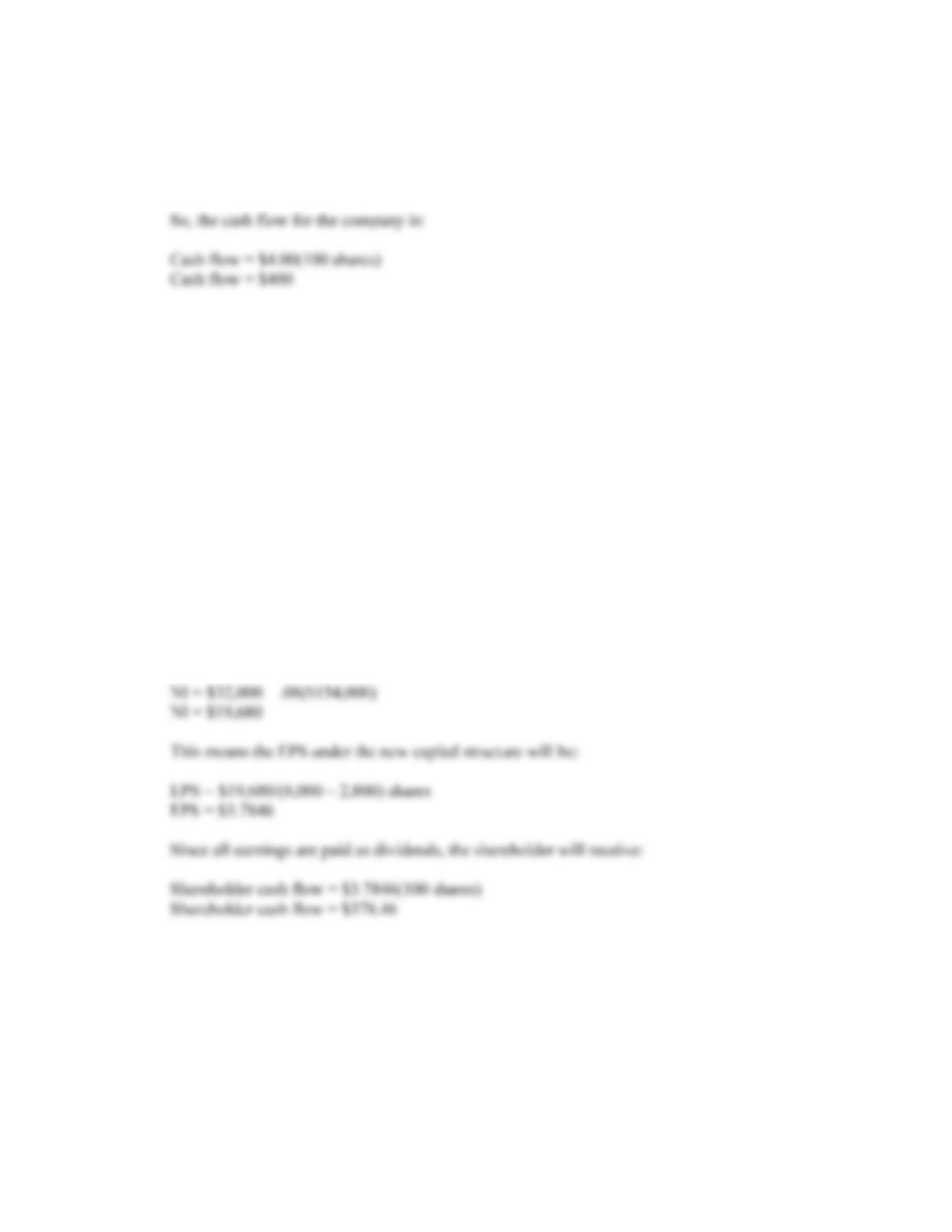CHAPTER 16 B-283
8. a. The earnings per share are:
EPS = \$32,000/8,000 shares
EPS = \$4.00
b. To determine the cash flow to the shareholder, we need to determine the EPS of the firm under the
proposed capital structure. The market value of the firm is:
V = \$55(8,000)
V = \$440,000
Under the proposed capital structure, the firm will raise new debt in the amount of:
D = 0.35(\$440,000)
D = \$154,000
in debt. This means the number of shares repurchased will be:
Shares repurchased = \$154,000/\$55
Shares repurchased = 2,800
Under the new capital structure, the company will have to make an interest payment on the new
debt. The net income with the interest payment will be:
c. To replicate the proposed capital structure, the shareholder should sell 35 percent of their shares, or
35 shares, and lend the proceeds at 8 percent. The shareholder will have an interest cash flow of:
Interest cash flow = 35(\$55)(.08)
Interest cash flow = \$154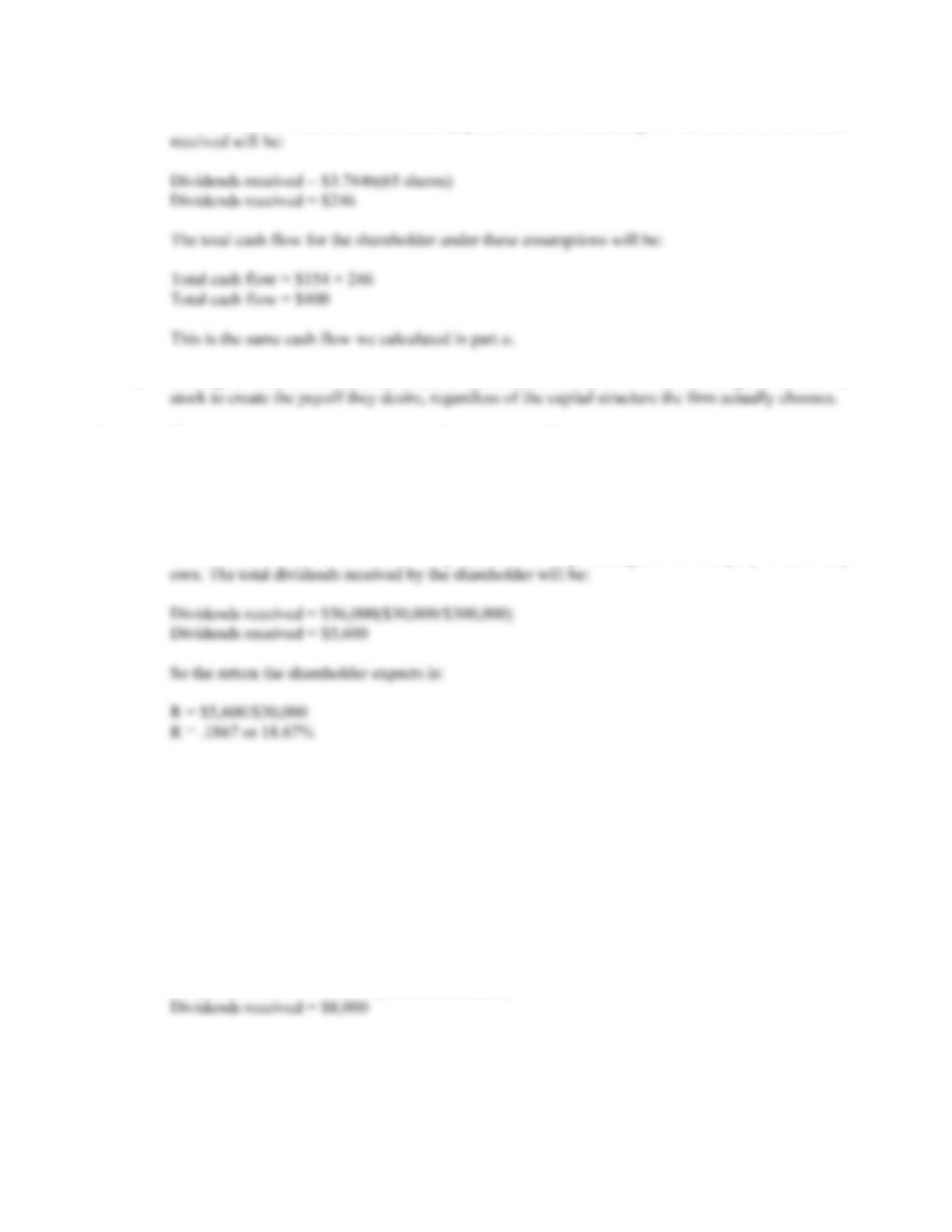B-284 SOLUTIONS
The shareholder will receive dividend payments on the remaining 65 shares, so the dividends
d. The capital structure is irrelevant because shareholders can create their own leverage or unlever the
9. a. The rate of return earned will be the dividend yield. The company has debt, so it must make an
interest payment. The net income for the company is:
NI = \$80,000 – .08(\$300,000)
NI = \$56,000
The investor will receive dividends in proportion to the percentage of the company’s share they
b. To generate exactly the same cash flows in the other company, the shareholder needs to match the
capital structure of ABC. The shareholder should sell all shares in XYZ. This will net \$30,000.
The shareholder should then borrow \$30,000. This will create an interest cash flow of:
Interest cash flow = .08(–\$30,000)
Interest cash flow = –\$2,400
The investor should then use the proceeds of the stock sale and the loan to buy shares in ABC. The
investor will receive dividends in proportion to the percentage of the company’s share they own.
The total dividends received by the shareholder will be: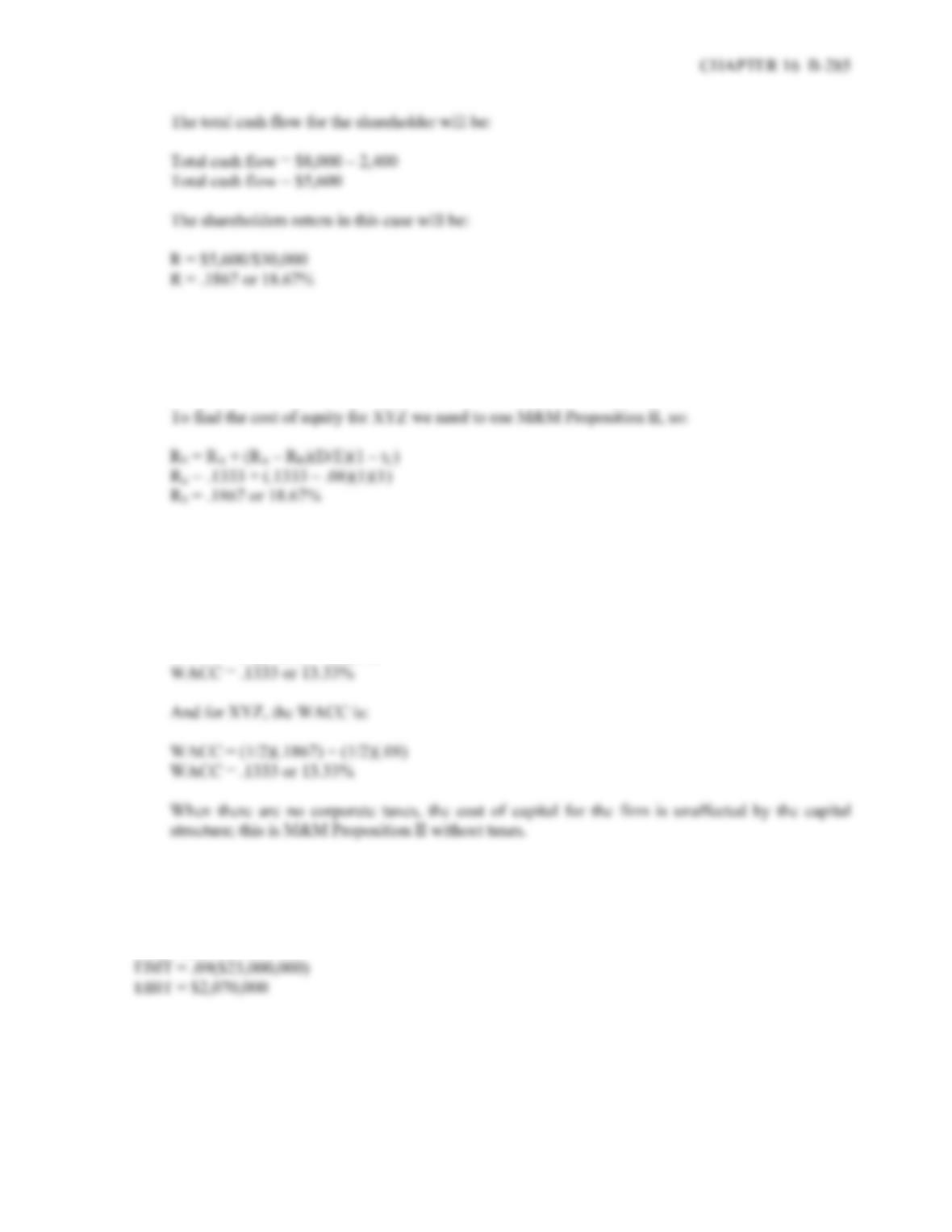c. ABC is an all equity company, so:
R
E = RA = \$80,000/\$600,000
R
E = .1333 or 13.33%
d. To find the WACC for each company we need to use the WACC equation:
WACC = (E/V)RE + (D/V)RD(1 – tC)
So, for ABC, the WACC is:
WACC = (1)(.1333) + (0)(.08)
10. With no taxes, the value of an unlevered firm is the interest rate divided by the unlevered cost of equity,
so:
V = EBIT/WACC
\$23,000,000 = EBIT/.09